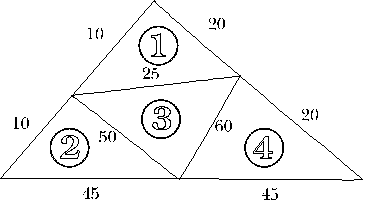#### You may also like### Two Cubes

Two cubes, each with integral side lengths, have a combined volume equal to the total of the lengths of their edges. How big are the cubes? [If you find a result by 'trial and error' you'll need to prove you have found all possible solutions.]### Rationals Between...

What fractions can you find between the square roots of 65 and 67?### Square Mean

Is the mean of the squares of two numbers greater than, or less than, the square of their means?

# Tet-trouble

##### Age 14 to 16Challenge Level

The following solution was sent in by Becki from the Mount School, York. Becki uses The Triangle Inequality. Andy from the Garden International School used the same method .

It is definitely impossible to have a tetrahedron which has edge lengths of 10, 20, 30, 40, 50 and 60 units.

This is because to produce a triangle two sides have to be bigger (when added together) than the third. So this tetrahedron won't work because the 10 is too small to make a triangle. e.g. 10, 20, 30

• 20 + 30 > 10 (OK)
• 10 + 30 > 20 (OK)
• but 10 + 20 = 30 so the triangle becomes a straight line.

10 will not go with any other two numbers in the above list.

However it is possible to make a tetrahedron which has lengths 10, 20, 25, 45, 50 and 60 units. The reason is that the numbers are big and small enough to fit the rule of triangles (the sum of two sides bigger than the third) Here is my net of this tetrahedron to explain my answer (not to scale!)Triangle 1 10 + 25 > 20 Triangle 2 10 + 50 > 45 10 + 20 > 25 10 + 45 > 50 20 + 25 > 10 50 + 45 > 10 Triangle 3 50 + 60 > 25 Triangle 4 60 + 45 > 20 25 + 60 > 50 20 + 60 > 45 25 + 50 > 60 20 + 45 > 60

So all four faces are fine, so it can make a tetrahedron.